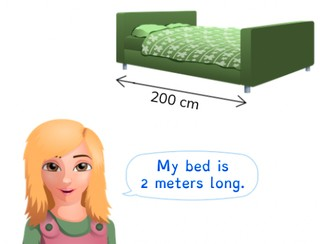Converting cm and m

# Converting cm and m

Students learn to convert cm and m.

No account needed.8,000 schools use Gynzy92,000 teachers use Gynzy1,600,000 students use Gynzy

## General

The students learn to convert centimeters to meters and vice versa.

## Standards

CCSS.Math.Content.4.MD.A.1

## Learning objective

Students will be able to convert centimeters (cm) to meters (m) and vice versa.

## Introduction

There are a few statements on the interactive whiteboard about meters. The students think about whether the statement is true or false. When they think that the statement is true, they stand up. If they think the statement is false, they remain seated. You will also do this with statements about centimeters.

## Instruction

You explain using a diagram of the metric system that you take two jumps to the left if you want to convert centimeters to meters. You also state that you divide the centimeters by one hundred, because you take two jumps dividing by ten and 10 × 10 = 100. You then show this with the problems in which you must convert centimeters to meters. After this you explain that when you don't have an exact number of meters, you will have a few centimeters left over. By first checking how many meters you have and then seeing how many centimeters you have left over, you can calculate how many meters and centimeters it is. You then have the students drag the numbers to the correct boxes. Next, you explain that when you want to convert meters to centimeters, you take two jumps to the right, so you multiply by one hundred. You show this through converting various lengths in meters to centimeters. Then you explain that when you don't have an exact number of meters, you first convert the meters to centimeters and then add the remaining centimeters. The students drag the numbers to the correct boxes. Finally you are going to solve story problems. Discuss the steps that you must follow when solving this type of story problem.

To check whether the students can convert centimeters to meters and vice versa, you can ask the following questions:
- How many centimeters are in one meter?
- What do you do when you convert centimeters to meters?
- What do you do when you convert meters to centimeters?

## Quiz

The students test their understanding of converting centimeters to meters, and meters to centimeters, through ten exercises. Some of these exercises are story problems.

## Closing

Repeat the goal of the lesson and the importance of it. Next there are a number of problems on the interactive whiteboard, for which the students must convert meters to centimeters and vice versa. You can have the students solve these and then reveal the answers by removing the covers.

## Teaching tips

When students have difficulty with this learning goal, you can give them a diagram of the metric system of length, in which you only have centimeters and meters with an arrow for ÷100 and an arrow for ×100.

## Instruction materials

You may use a diagram of the metric system.

### The online teaching platform for interactive whiteboards and displays in schools

• Save time building lessons

• Manage the classroom more efficiently

• Increase student engagement